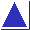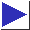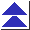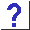# comp.lang.c FAQ list ·Question 3.17

Q: I have some code containing expressions like

`a ? b = c : d`
and some compilers are accepting it but some are not.

A: In the original definition of the language, = was of lower precedence than ?:, so early compilers tended to trip up on an expression like the one above, attempting to parse it as if it had been written

```	(a ? b) = (c : d)
```
Since it has no other sensible meaning, however, later compilers have allowed the expression, and interpret it as if an inner set of parentheses were implied:
```	a ? (b = c) : d
```
Here, the left-hand operand of the = is simply b, not the invalid a ? b. In fact, the grammar specified in the ANSI/ISO C Standard effectively requires this interpretation. (The grammar in the Standard is not precedence-based, and says that any expression may appear between the ? and : symbols.)

An expression like the one in the question is perfectly acceptable to an ANSI compiler, but if you ever have to compile it under an older compiler, you can always add the explicit, inner parentheses.

References: K&R1 Sec. 2.12 p. 49
ISO Sec. 6.3.15
Rationale Sec. 3.3.15